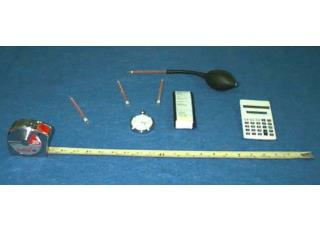DownloadDownload Presentation14 sec 12 sec 13 sec 16 sec

14 sec 12 sec 13 sec 16 sec

Télécharger la présentation14 sec 12 sec 13 sec 16 sec

- - - - - - - - - - - - - - - - - - - - - - - - - - - E N D - - - - - - - - - - - - - - - - - - - - - - - - - - -
Presentation Transcript

1. Low Velocity Air Measurements (timed smoke clouds) 14 sec 12 sec 13 sec 16 sec 13 sec 14 sec 14 sec 15 sec 14 sec 14 sec 13 sec 15 sec 13 sec 15 sec 13 sec 15 sec The time it took the leading edge of the smoke cloud to travel 10 feet was recorded (4 times in each quadrant). H + H + H 1 2 3 Average H = 3 8.0’ 8.2’ 7.9’ 19.7’ The dimensions of the mine entry were measured. (sum of all times) Average time = A = W x H (number of times) (distance) 60 sec x x 0.9 Average V = (average time) 1 min

2. Low Velocity Air Measurements (timed smoke clouds) 8.0’ 8.2’ 7.9’ 19.7’ The dimensions of the mine entry were measured. 13 sec 14 sec 14 sec 15 sec 14 sec 12 sec 13 sec 16 sec H + H + H 1 2 3 13 sec 15 sec 13 sec 15 sec 14 sec 14 sec 13 sec 15 sec Average H = 3 The time it took the leading edge of the smoke cloud to travel 10 feet was recorded. 8.0’+8.2’+7.9’ Average H = = 8.0’ 3 (223 sec) Average time = = 13.9 sec A = W x H (16) A = 19.7’ x 8.0’ (10’) 60 sec x x 0.9 Average V = A = 158 sq.ft. (13.9 sec) 1 min Average V = 39 fpm q = V x A q = 39 fpm x 158 sq.ft. = 6162 cfm

3. Low Velocity Air Measurements (timed smoke clouds) H + H + H 1 2 3 Average H = 3 (sum of all times) (number of times) (distance) 60 sec x x 0.9 Average V = (average time) 1 min 14 sec 13 sec 14 sec 15 sec no movement 4.5’ 4.7’ 4.4’ 20.4’ 15 sec 14 sec 13 sec 14 sec no movement The dimensions of the mine entry were measured. The time it took the leading edge of the smoke cloud to travel 5 feet was recorded (4 times in each quadrant). Average time = A = W x H

4. Low Velocity Air Measurements (timed smoke clouds) 4.5’ 4.7’ 4.4’ 20.4’ The dimensions of the mine entry were measured. 14 sec 13 sec 14 sec 15 sec no movement 15 sec 14 sec 13 sec 14 sec no movement H + H + H 1 2 3 Average H = The time it took the leading edge of the smoke cloud to travel 5 feet was recorded. 3 4.5’+4.7’+4.4’ Average H = = 4.6’ (112 sec) 3 Average time = = 14 sec (8) A = W x H (5’) A = 20.4’ x 4.6’ 60 sec x x 0.9 Average V = (14 sec) 1 min A = 93.8 sq.ft. (93.8 sq.ft.) Effective Area = Average V = 19 fpm 2 Effective Area = 47 sq.ft. q = V x A q = 19 fpm x 47 sq.ft. = 893 cfm